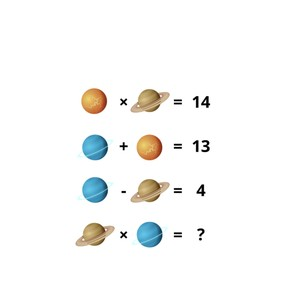Math Puzzles

# Math Puzzles

Practice your problem-solving skills with these challenging math puzzles!8,000 schools use Gynzy92,000 teachers use Gynzy1,600,000 students use Gynzy

## General

Students practice their problem-solving skills with these challenging math puzzles! Each image represents a number. Find the value of the individual pictures by solving the mathematical equations.

These pre-algebraic puzzles that use substitution help students to perform basic functions and encourages their problem-solving skills. They use abstract reasoning and must find patterns to solve the problems given.

## Standards

Math Practice 1, Math Practice 7, Operations & Algebraic Thinking K-5, Expressions & Equations 6-7

## Learning Objective

Students will be able to apply knowledge and recognize patterns to solve the math puzzles and find the value of different images in equations.

## Tips

Select a math puzzle to solve with the class. The puzzles are increasingly difficult. Give students time to work individually, in pairs, or in groups to determine the solution to the given problem. Discuss answers as a class and have students explain their thinking before revealing the answer to the class.

### The online teaching platform for interactive whiteboards and displays in schools

• Save time building lessons

• Manage the classroom more efficiently

• Increase student engagement